https://calib.io/blogs/knowledge-base.atom calib.io - Knowledge Base 2022-08-04T12:04:05+02:00 calib.io https://calib.io/blogs/knowledge-base/understanding-reprojection-errors 2022-08-04T12:04:05+02:00 2022-08-04T13:01:59+02:00 Understanding Reprojection Error Eyþór Rúnar Eiríksson //

In camera calibration, the reprojection error (RPE) is of central importance. What exactly is it and how can we interpret it? This article aims to shed some light on this important, and sometimes slightly misunderstood topic.

### What is the reprojection error?

Formally, a reprojection error is a:

2d vector of the difference between measured and projected point

Below you can see how Calibrator visualises both the measured (green cross) and the projected (red circle) point. Because the individual error tends to be much smaller than a single pixel, Calibrator draws the reprojection error as a 10 x scaled version using a red line.Let call the measured point by $p_{ij}$. Here $i$ denotes the point's unique id on the calibration board and $j$ the pose/image number. In the camera calibration setting, its' coordinates are found using a feature detection algorithm - e.g. chessboard detector, circle grid detector, etc. The detector's job is to find the calibration target in the image. This step is usually followed by sub-pixel optimisation where the surrounding image values are used to actually determine the feature's position with accuracy well below 1px.

The projected point $\breve{\vec{p}}_{ij}$ is obtained by taking the $i$-th point nominal calibration board coordinates, applying the $j$-th pose's extrinsic transformation, and lastly applying the camera's projection mapping. The extrinsic transformation is a 3D roto-translation which related the calibration target's local coordinate frame in the specific pose/shot to the camera's coordinate frame. The camera's projection mapping depends on which (mathematical) camera model is being used. Most common camera models perform perspective projection combined with non-linear lens distortion.

The objective function being minimised in camera calibration is:

$$\sum_i{\sum_j{||\vec{p}_{ij} - \breve{\vec{p}}_{ij}||^2}} \quad ,$$

- that is the sum of squares of the norm/length of all re-projection errors is minimised. In other words, the calibration process adjusts all extrinsics and camera parameters such that the points project as close to where they were measured as possible.

Most commonly, the root-mean-square of all reprojection error norms is reported by calibration software, and called simply the reprojection error.

$$\textrm{RPE}_{\textrm{RMS}} = \sqrt{1/N \cdot \sum_i{\sum_j{||\vec{p}_{ij} - \breve{\vec{p}}_{ij}||^2}}}$$

Beware - the mean or median of reprojection error are also sometimes reported, and nomenclature is not $100\%$ consistent in the literature.

$$\textrm{RPE}_{\textrm{mean}} = 1/N \cdot \sum_i{\sum_j{||\vec{p}_{ij} - \breve{\vec{p}}_{ij}||}}$$

$$\textrm{RPE}_{\textrm{median}} = \textrm{median}(||\vec{p}_{ij} -\breve{\vec{p}}_{ij}||)$$

Especially in the case of severe outliers, these quantities could differ quite significantly. In conclusion - technically the reprojection error is a single vector quantity, but in most settings a statistic (RMS) of all reprojection errors is meant with reprojection error.

### What are the Error Sources?

Why does the RPE never become exactly zero? There is a few sources which contribute to this error that cannot be fully eliminated.

First is feature noise - aka non-perfect detection of the saddle points or circles on a calibration target. It can be further broken down into image noise (comprised of dark current, shot noise and quantisation noise) and detector error/bias.

Secondly, the mathematical camera model might not be flexible enough to accurately model the complex optical behaviour of the lens and sensor.

Thirdly, the calibration target which normally is assumed perfect, might not be. For planar boards, stiffness, rigidity and temperature stability are crucial, and if not good enough will lead to higher RPE.

Lastly, the initial guess used for calibration may not have been in the vicinity of the global minimum, which leads to a non-optimal solution.

Error sources can be unbiased and stochastic, or biased, which could lead the calibration process off-track. The important question is whether the errors are correlated with the camera parameters or not.

As an example, while image noise does contribute to RPE, its stochastic nature would leads to a zero net-effect on the solution if enough images and feature coordinates are used.

A calibration target printed in a normal laser printer could easily have a bias with checker being non-square, etc. This effect will contribute to RPE and most likely bias the found solution.

### What does the RPE tell us?

Now that we have clearly defined the reprojection error and analysed its' sources, we can understand what meaning it really bears.

First of all, note that the units of RPE is pixels. Hence, with higher resolution camera sensor and smaller pixels, we'd automatically expect higher RPE. Multiplying the RPE by pixel size of the given sensor (usually few micrometers), you get a somewhat comparable quantity which using trigonometry can be used to derive the equivalent error at the working distance.

As such, the RPE gives an indication of how accurately we are able to detect features and how accurately we project 3D coordinates.

However, the RPE of the calibration images used is a training error - it was the lowest number achieved on the given data. As common in statistics, we risk overfitting by focusing on the RPE alone. As an example, using very few calibration images, covering only a small part of the image, and with many calibration parameters active (flexible camera model), we most likely achieve a very low RPE. Closer analysis of the covariance structure allows us to estimate the much more conducive test error.

With Calibrator, we can analyse the projection and triangulation errors, and hence quantify our calibration with statistics that are much more meaningful and comparable than the RPE, and tell us how accurate we can expect the given camera setup to be in real-world units.

]]>
https://calib.io/blogs/knowledge-base/understanding-parameter-uncertainty 2022-06-17T16:26:22+02:00 2022-07-22T20:52:33+02:00 Understanding Parameter Uncertainty Jakob W.

Camera calibration generally aims to fit a suitable mathematical model to data in order to geometrically characterize one or more camera/lens-combinations.

Many users aim to lower the re-projection error (RPE) as much as possible. While this is, indeed, desirable in most cases, we advocate to judge calibration quality on more than the obtained RPE. First and foremost, parameter uncertainties play a crucial role for the camera system’s real world performance.

Let's dive deeper into this topic using a simpler data-fitting example which should unveil the importance of uncertainty in data fitting.

### A Fitting Problem

In the below figure, we have plotted a single sinusoidal half-wave which serves as our ground truth function that we aim to describe as faithfully as possible. (Note that in contrast to a camera model which maps $R^3 \rightarrow R^2$, this function is just $R \rightarrow R$, but the concepts apply irregardly.)Unfortunately, we do not know this real function in a calibration setting. Instead we would have a number of sampled values which are affected by noise and possibly bias. In camera calibration the sources of noise and bias include sensor dark current, shot noise, quantisation noise, defocus, etc. Below we have sampled data with noise distributed according to the normal distribution $\mathcal{N}\left(0, 0.05 \right)$.In this case, we have 8 data points and our aim is to fit a function, which hopefully resembles the true underlying function as good as possible. For technical reasons, we might only have samples from the central part of the domain, which in this case is the interval $[0; \pi]$. This is a very typical case also in camera calibration, when e.g. chessboard detection might require that the entire target is inside the image, leaving the outer image regions un- or under-sampled.

### Parabolic Fit

An easy model to fit using ordinary least squares would be a parabola (a 2nd degree polynomial) $y = a x^2 + b x + c$. In the figure below the best fit parabola is shown, along with the fit's $95\%$ confidence bands.It can be observed that the true underlying model was reconstructed reasonably, but especially at the domain boundaries, where no data is given, the true function actually falls outside of the confidence region. Further, the confidence region has considerable width over the entire function meaning that a new sampling of data points will likely lead to a parabola with quite different coefficients.

We have computed a root-mean-square error (RMSE) of $0.026$, which is the root of the mean of the squares of the vertical red error-bars. This is again analogous to camera calibration, where usually the RMS of reprojection errors is reported.

The found best-fit parabola is:

$y = -0.12 x^2 + 1.42 x - 0.46$

We are also able to estimate the covariance of the paramers at our solution:

$\left[\begin{array}{*{3}c} C_{a,a} & C_{a,b} & C_{a,c} \\ C_{a,b} & C_{b,b} & C_{b,c} \\ C_{a,c} & C_{b,c} & C_{c,c} \\ \end{array}\right]$ = $\left[\begin{array}{*{3}c} 0.0036 & -0.0048 & 0.0014 \\ -0.0048 & 0.0074 & -0.0023 \\ 0.0014 & -0.0023 & 0.0007 \\ \end{array}\right]$

Square roots of the diagonal entries give us the standard deviation of each of the model parameters:

$\sigma_a = \sqrt{0.0036} = 0.06$

$\sigma_b = \sqrt{0.0074} = 0.08$

$\sigma_c = \sqrt{0.0007} = 0.03$

This tells us that there is considerable uncertainty in all of the parameters. Off-diagonal entries are also non-negligible which means that certain combinations of parameters have high uncertainty. Taking $C_{a,b} = -0.0048$ as an example, increasing $a$ a little while decreasing $b$ a little would yield a very similar RMSE value.

Note that the correlation $\rho(i, j) = C_{i,j}/(\sqrt{C_{i,i}} \sqrt{C_{j,j}})$ between parameters might be easier to interpret as it normalizes these values to between $-1$ and $1$.

### Increasing Model Flexibility

In our hunt for low RPE values, we might consider increasing the degree of the fitting polynomial. This would correspond to adding more parameters to our model (think $k_3, k_4, k_5, ...$ in the OpenCV distortion model). Below is a 5th-order polynomial fit along with its $95\%$ confidence band.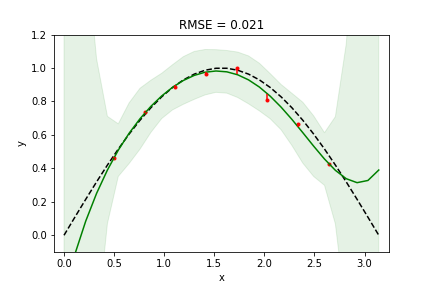Indeed we achieved a lower RPE value (from $0.026$ down to $0.021$), which a more flexible model would most always yield. However, at the right domain boundary we see our model diverting a lot from the truth. In addition, confidence bands are much wider, so we can't expect very consistent results when re-sampling. In statistics, this situation is called overfitting - there is a discrepancy between the model's flexibility and the amount of data to constraint the fit. In fact, since our noise has a standard deviation of $0.05$, that is the RMSE that we should expect to achieve.

In this case, the found polynomial coefficients (along with standard errors) are:

$a: -0.351 \pm 1.134$

$b: 2.399 \pm 4.911$

$c: -2.050 \pm 7.688$

$d: 1.253 \pm 5.539$

$e: -0.473 \pm 1.863$

$f: -0.067 \pm 0.237$

Standard errors are very high and many of these parameters are also heavily correlated. This gives rise to the very wide confidence bands above.

• Increasing model flexibility is not always warranted. Beware of over-fitting.
• Judging the fit's quality by its RMSE alone is not sufficient.

### Increasing the amount of data

With the last result in mind, one approach to avoid the overfitting problem could be to sample more data. In camera calibration terms, the number of target observations could be increased, or a calibration target with more visual features be used.

Below we have increased the number of samples to 50.We notice that confidence in the model has indeed increased significantly, but only in the region supported by data. RMSE has actually increased, but it was unnaturally low before due to the overfitting situation.

• Overfitting can be combated/avoided by sampling enough data.
• Higher-order polynomial models can "explode" outside regions with data support. (OpenCV users: beware of the higher-order polynomial distortion coefficients $k_2, k_3, k_4, ...$.)

### Adding boundary data

We still have low confidence in our model near the domain boundaries, so let's revert to the 2nd-order polynomial (parabolic fit) and investigate what happens if a few data points are added in these regions: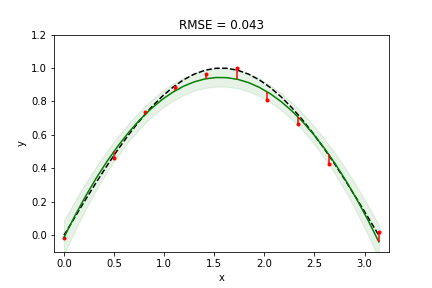RMSE has increased, but our fit is actually quite good across the entire domain.

• Samples from domain boundary regions help to constraint the model well.

### Modifying the model

So far, we have looked at polynomial models. Perhaps another mathematical model is better at describing the data. It might also work better in those regions where we do not have any information.

Below we have switched to a sinusoidal function $a \cdot \sin \left( w \cdot x \right ) + o$. Since we know that the true model is sinusoidal in nature, this model should fit really well.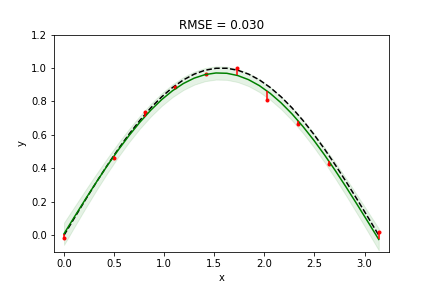And indeed it does. A result quite close to the ground truth with narrow confidence regions, even with comparatively little data.

Combining more data sampling and good coverage should yield even better results. Below we use the same sinusoidal model, but sample 50 values evenly spaced across the domain:By sampling enough data from the entire domain and choosing a function that is most capable of modelling the underlying true function with few parameters, we are able to achieve a very accurate and robust result.

Let's plot the obtained RMSE as a function of the number of samples (spaced uniformly on the interval $[0; \pi]$ with noise distribution $\mathcal{N}\left(0, 0.05 \right)$).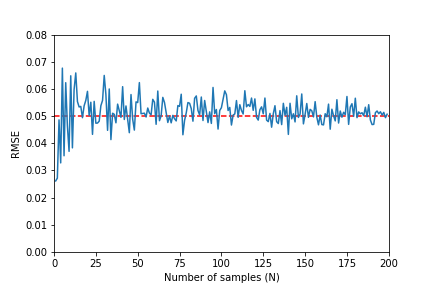As predicted, RMSE converges to the standard deviation of our the noise. A value clearly below it does not represent a good fit, but rather an overfitting situation.

### What This Means for Camera Calibration

Above we have explored a $R \rightarrow R$ function, as we can easily plot it and investigate different fits. However, everything that holds for data fitting here also applies to camera calibration which is just a higher dimensional data fitting problem.

In camera calibration we must also use the most suitable matematical model and use enough data to make all parameters well determined with as little standard error as possible. In addition, covariances/correlations should also be low. Similar to how confidence bands aid in the interpretation of covariance, we can propagate the uncertainty in calibration parameters to the image plane and visualize 95% confidence ellipses on the image plane. The screenshot below shows how Calibrator visualizes this result. The software also reports the RMS of simulated errors, which gives us an unbiased estimate of the true validation error.Taking things one step further, we might be interested in what the given uncertainty means for the precision of point triangulation in a multi-camera system. This given an easy to interpret value in units of meter/millimeters and reflects the benchmark that most user actually care about. Below is the "Triangulation error" view of Calibrator.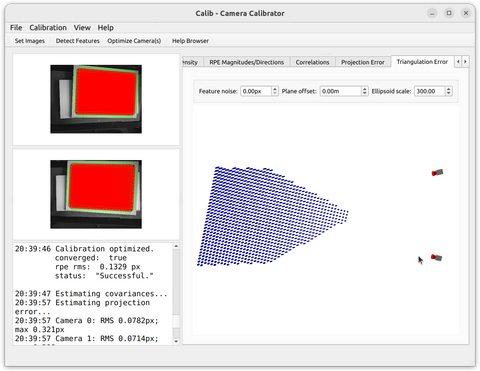To summarize:

• RMSE values should never stand alone as calibration success criteria.
• Using fewer parameter in the camera model and including more observations aids in avoiding overfitting.
• Confidence regions for individual parameters and their propagation into e.g. triangulation are important measures of calibration quality.

Calib.io's Camera Calibrator application lets you investigate all aspects of camera calibration errors and uncertainties. Standard errors, parameter covariances and triangulation errors are the camera calibration equivalents of confidence bands. It is crucial to take all these aspects into account for accurate camera calibration.

Thanks for helping us improve camera calibrations world wide. Your questions and comments are appreciated.

]]>
https://calib.io/blogs/knowledge-base/calibration-target-substrates-101 2021-05-20T15:18:28+02:00 2022-06-17T15:03:00+02:00 Exploring Calibration Target Substrates Eyþór Rúnar Eiríksson More

]]>

Many applications can make due with our standard Alu/LDPE composite substrate available on our store. However, we have various options and manufacturing processes to select from when it comes to special applications. Let's take a closer look at the substrates used in previous customer projects at Calib.io:

• Standard Aluminium/Low Density Polyethylene composite.
• Lambertian Substrate
• Honeycomb Aluminium
• Uni-Directional Carbon Fiber
• Glass (float glass, soda lime or quartz)
• Ceramic
• Passive Thermal Substrates
• Solid Metal (Steel, Aluminium)
• Retro Reflective Substrate
• Acryllic

In the next sections we will go over the pros and cons of each material option starting with our standard.

## Aluminium/LDPE

This is the standard substrate we offer in our webshop.

The substrate is a composite of aluminium and Low-density polyethylene (LDPE). The LDPE, which accounts for the majority of the thickness, is sandwiched between two thin sheets of aluminium (0.3 mm each). This substrate provides a nice compromise between stiffness and weight. The patterns are transferred to the composite material using a specialised precision direct UV (Ultra-Violet) printing process resulting in a ultra durable finish. As a result it is lightweight, low cost, robust and flat. It is easily cut and machinable if mechanical features are required. We can supply targets in the following thicknesses:

 Thickness [mm] Weight [kg/m$^2$] Rigidity E·I[kNcm$^2$/m] 2 2.90 345 3 3.80 865 4 4.75 1620 6 6.60 3840

In all cases we recommend the 6 mm thickness as it is most rigid and likely to maintain its flatness. The thinner the substrate is the less area we would recommend before a special frame or fixture is required to increase stiffness. In the 6mm case, anything above 800x600 mm will require our rigid frame solution so that flatness can be maintained (See example here) . Alternatively, we would recommend our thicker and lighter Honeycomb substrates ( See below )

Some notable features of this substrate are:

• Water and sea submersible, for long periods of time. Excellent candidate for underwater calibration.
• Recommended for the harshest environmental conditions, both indoor and outdoor.
• Cleanable with a wet rag or soapy water.
• Lightweight
• Dual sided prints possible.
• Optimised for visible light.

As for optical properties, the substrate offers semi-matte characteristics and has a "gloss value" of approximately 40 @ 60deg in the white areas and 5 @ 60deg in the black. For applications using strong illumination, some care needs to be taken to avoid specular reflections. If this is unavoidable, then we recommend our Lambertian substrate.

For applications in the near infrared and above (e.g 850 nm), the substrate exhibits more specular reflection and a slightly degraded contrast. In this case we would also recommend the Lambertian substrate.

## Lambertian

Our Lambertian substrate exhibits Lambertian Reflectance properties, where in the ideal case the reflected light is diffuse and no specular reflections (mirror reflections) occur. This is possible due to the substrates fine micro-structure which scatters the light in random directions in a diffused fashion. The following image shows a comparison between our lambertian substrate and our Alu/LDPE substrate: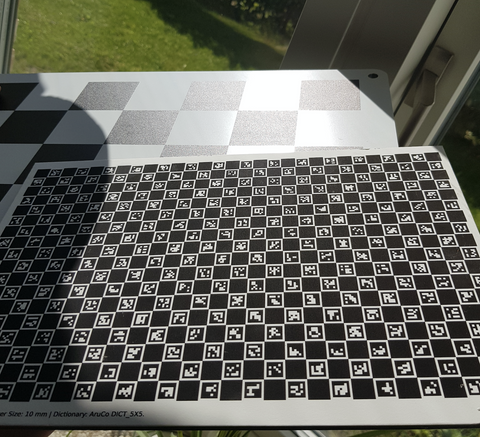As it can be seen from the picture, the lambertian substrate (CharuCo target) has no specular reflections and significantly outperforms the standard substrate. The Lambertian substrate has a gloss 60 value of only 3.

The substrate is in all cases laminated to a rigid backing. Our most popular backing material is aluminium/LDPE. The honeycomb, glass, thermal and solid metal substrates can also be used depending on the application requirements.

Due to the fine microstructure on the Lambertian substrate, it is quite sensitive to damage and not nearly as robust as the Alu/LDPE substrate. Therefore extra precautions and care need to be taken in order to not damage the target. We therefore only recommend this target for lab environments.

## Honeycomb Aluminium

The honeycomb substrate consists of two 1 mm thick aluminium sheets which sandwitch a honeycomb aluminium structure. This material is known for its superior stiffness to weight ratio and flatness retention which can be seen in the following table:

 Thickness [mm] Weight [kg/m$^2$] Rigidity E·I[kNcm$^2$/m] 6 4.7 7100 10 5.0 21900 15 6.7 75500 20 7.0 138900 25 7.3 221600

For large targets, the 25 mm option would be an excellent choice over the 6 mm Alu/LPDE substrate as the weight is comparable but rigidity is nearly 58x greater.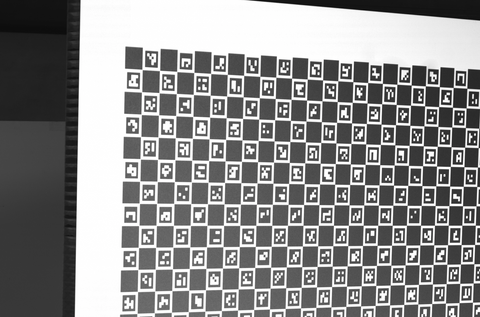The above image shows a large >1m x 1m charuco target at 25 mm thickness without edge banding. ABS or Aluminium edge banding can be applied upon request.

## Uni-Directional Carbon Fiber

The carbon fiber substrate is an excellent choice for calibration targets as offers an excellent stiffness to weight ratio. This substrate is available in 3, 4, 5, 6 and 7 mm thicknesses. For targets over 800x600 mm we would strongly recommend using an aluminium frame to improve rigidity. It is solely available as a substrate for our lithography process which offers accuracy in the micrometer range. If the application is less demanding then we would recommend our standard Alu/LDPE substrate. The "Gloss 60" value is 3.## Glass / Float Glass / Quartz / Soda Lime

Glass is commonly used for its excellent flatness. We can provide this substrate in various thicknesses as a backing material for our Lambertian substrate, direct UV print or lithography processed paint if micrometer precision is required. This substrate is not recommended for large targets and shipping costs will also be higher. These substrates can also be made without a flood color, making them transparent and suitable for backlit operations.

## Ceramic

We offer ceramic substrates for precision lithography processed targets for the most demanding applications. The process deposits 100nm blue toned matte chrome coating ontro a white ceramic backing, capable of obtaining accuracy in the micrometer range. Substrates are extremely flat and can be sourced in 0.635mm and 1 mm thicknesses. Due to it's thickness there is an upper bound to how large these can be. Largest 0.635 mm is 120x120 and the 190x139 mm for the 1 mm.## Passive Thermal Substrates

For short and long wave infrafred (SWIR and LWIR) calibration we would recommend our passive thermal substrates. The core feature of the substrate is that it has high thermal capacity and low thermal conductivity making it the ideal choice for thermal camera calibration. An added benefit is that it can also be used for standard visible light cameras which is essential for multi sensor calibration.

In operation, our customers heat up the target using an external heating source, such as a heating lamp, and measure the emissivity of the radiated heat. The difference in emissivity for black and white region is significant enough such that a good contrast is obtained:Currently, two types of substrates are available. One is polymer based and the other a composite of our Lambertian substrate and medium density fibreboard.

Polymer based: This substrate goes through our standard UV print process and is extremely robust and offers superior emissivity contrast. It is ideal for harsh indoor/outdoor conditions and may be cleaned with a wet cloth and soapy water. It comes in a 19 mm thickness to ensure its rigidity. One downside is when using constant active heating this target can be slightly reflective and if this is the case we would recommend adding our Lambertian substrate using the Polymer backing or the medium density fibreboard

Medium Density Fibreboard: This substrate we use as a backing to our Lambertian substrate. It offers slightly reduced emissivity contrast at the cost of superior optical properties due to the lambertian substrate. However, this combination is easily damaged and sensitive. It is therefore only recommended for lab settings. Available at 19mm thickness in black backing color.

## Solid Metal (Steel, Aluminium)

Various other metal substrates can be used if the applications calls for it. These substrates would be ideal if precision mechanical features are required. An example of this would be this 3D calibration target, machined out of aluminium, andozied and engraved.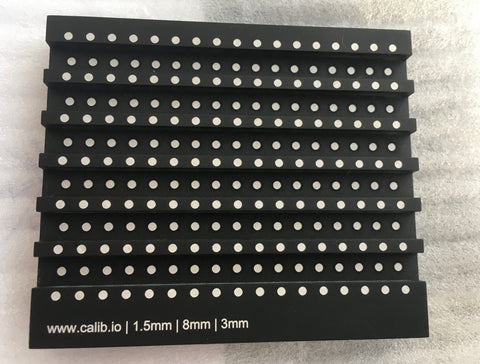Precision CNC maching and surface grinding can be performed to obtain superior tolerances.

## Retro-Reflective

We carry special retro reflective substrates which we can laminate to any backing of your choice. The black portions of the pattern are printed using our standard UV process. This substrate is suitable for applications using active illumination close to the optical axis, requiring strong contrast.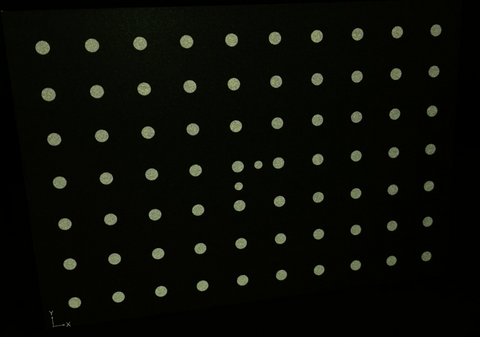## Acryllic Substrate

This substrate is available in 2, 3 and 4 mm thicknesses and can be used as a lightweight alternative to float glass for backlit applications with little to no risk of breaking. One benefit being that our particular substrate is 30% transparent which acts as a light diffuser. It may not be as rigid as e.g glass but it won't break when dropped. We exclusively use a UV print manufacturing process which makes this an affordable, robust and reliable transparent option.

# Other substrates

Don't see a substrate listed here that you would like? Please get in contact with us and let us know! We would be happy to investigate the use of other alternative substrates  for your application.

]]>
https://calib.io/blogs/knowledge-base/calibration-best-practices 2018-11-15T17:15:00+01:00 2021-03-31T16:32:19+02:00 Calibration Best Practices Jakob W. Accurate calibration is of key importance for performance in most machine and computer vision tasks. The following lists our best practices which we have found through extensive experimentation and theoretical considerations.

1. Choose the right size calibration target. Large enough to properly constrain parameters. Preferably it should cover approx. half of the total area when seen fronto-parallel in the camera images.
2. Perform calibration at the approximate working distance (WD) of your final application. The camera should be focused at this distance and lens focus should be unchanged after calibration.
3. The target should have a high feature count. Using fine patterns is preferable. However, at some point detection robustness suffers. Our recommendation is to use fine pattern counts for cameras above 3MPx and if the lighting is controlled and good.
4. Collect images from different areas and tilts. Move the target to fully cover the image area and aim for even coverage. Lens distortion can be properly determined from fronto-parallel images, but focal length estimation is dependent on observing foreshortening. Include both frontoparallel images, and images taken with the board tilted up to +/- 45 degrees in both horizontal an vertical directions. Tilting more is usually not a good idea as feature localization accuracy suffers and can become biased.
5. Use good lighting. This is often overlooked, but hugely important. The calibration target should preferably be diffusely lit by means of controlled photography lighting. Strong point sources give rise to uneven illumination, possibly making detection fail, and not utilizing the camera's dynamic range very well. Shadows can do the same.
6. Have enough observations. Usually, calibration should be performed on at least 6 observations (images) of a calibration target. If a higher order camera or distortion model is used, more observations are beneficial.
7. Consider using uniquely coded targets such as CharuCo boards. These allow you to gather observations from the very edges of the camera sensor and lens, and hence constrain the distortion parameters very well. Also, they allow you to collect data even when some of the feature points do not fulfil the other requirements.
8. Calibration is only as accurate as the calibration target used. Use laser or inkjet printed targets only to validate and test.
9. Proper mounting of calibration target and camera. In order to minimize distortion and bow in larger targets, mount them either vertically, or laying flat on a rigid support. Consider moving the camera instead of the target in these cases instead. Use a quality tripod, and avoid touching the camera during acquisitions.
10. Remove bad observations. Carefully inspect reprojection errors. Both per-view and per-feature. If any of these appear as outliers, exclude them and recalibrate.
11. Obtaining a low reproduction error does not equal a good camera calibration, but merely indicates that the provided data/evidence can be described with the used model. This could be due to overfitting. Parameter uncertainties are indications of how well the chosen camera model was constrained.
12. Analyse the individual reprojection errors. Their direction and magnitude should not correlate with position, i.e. they should point chaotically in all directions. Calib.io's Camera Calibrator software provides powerfull visualizations to investigate the reprojected errors.

Following these practices should ensure the most accurate and precise calibration possible.

Have any questions, comments or additional insights? Post them below.

]]>
https://calib.io/blogs/knowledge-base/camera-calibration 2018-11-15T16:38:00+01:00 2022-08-04T10:54:21+02:00 Camera Calibration Explained Jakob W.

Camera calibration is the process of determining camera and lens model parameters accurately. With the common Brown-Conrady camera model, this amounts to determining the parameters of a suitable camera model, at least the focal length $f$, and possibly central point coordinates ($c_x, c_y$) and lens distortion parameters $\boldsymbol{k}$.

In the most common, offline calibration process, images are taken under specific constraints. The calibration object defines a world coordinate system such that 3D coordinates of the visual features are known. Most of these methods work by observing a calibration object with known visual features. This is preferred when full control over the calibration procedure is necessary and high accuracy is demanded.

Camera Model

In any camera calibration effort, it is crucial to select a suitable camera model, which neither under- nor over-parameterized the camera. More information on camera models is found in our article on the subject

Calibration Procedures

Many procedures for camera calibration have been proposed in literature. See e.g. Tsai's method  and Heikkilä and Silvén's . These procedures differ in the type of calibration object needed, and the derivation of an initial guess for the camera parameters and the following nonlinear optimization step. Probably the most popular of all procedures is Zhang's .

All of these methods should always be followed by non-linear optimisation (bundle adjustment) as they only return algebraic solutions and don't account for lens effects. They do however provide an initial guess which is required for the non-linear optimisation to converge.

Zhang's Method

A modern and popular method in the computer vision community is that of Zhang, which also is implemented in popular software libraries such as libCalib, OpenCV, Jean-Yves Bouguet's Camera Calibration Toolbox for Matlab and Matlab's Computer Vision Toolbox. Zhang's calibration routine relies on observations of a planar calibration board with easily recognizable features. It begins by neglecting lens-distortion, and relates the 2-D coordinates of these to the observed image coordinates projections by means of homographies. This allows to solve for the most important pinhole parameters and for the calibration plane extrinsics (the camera's position and orientation relative to the calibration board's coordinate system), by means of a closed form solution.

Tsai's method

In contrast to Zhang's methods, Tsai's does not formulate the relation between object and image points as a series of homographies. Instead, algebraic constraints are derived which lead to a stepwise procedure to incrementally eliminate the unknowns. The camera is modelled as a standard pinhole + a single radial distortion coefficient.

One advantage of Tsai's method over Zhang's is that it can find a solution for the model parameters also for non-planar calibration objects (e.g. step targets or V-shaped calibration rigs). For this reason, libCalib implements Tsai's method.

Following any of the initialisation methods above, non-linear optimisation is employed to refine the parameter estimates and to include additional camera parameters which cannot be solved for with those techniques (e.g. radial lens distortion parameters). This estimation problem can be seen as a particular case of bundle block adjustment (sometimes just bundle adjustment), and it aims to find the max-likelihood solution to minimise the average reprojection error under the assumption of Gaussian noise in feature detections.

The objective function to be minimized is the sum of squared reprojection errors, defined in the image plane:
$$\sum_i{\sum_j{||\vec{p}_{ij} - \pi(\vec{P}_j, \boldsymbol{K}, \vec{k}, \boldsymbol{R}_i, \vec{T}_i)||^2}} \quad ,$$
where $\pi(\vec{P}_j, \boldsymbol{K}, \vec{k}, \boldsymbol{R}_i, \vec{T}_i)$ is the projection operator determining 2-D point coordinates given 3-D coordinates and the camera parameters. $i$ sums over the positions of the calibration board and $j$ over the points in a single position. $\vec{P}_j$ are 3-D point coordinates in the local calibration object coordinate system, $\vec{P}_j = [x, y, 0]^\top$, and $\vec{p}_{ij}$ the observed 2-D coordinates in the camera. The per-position extrinsic $\boldsymbol{R}_i, \vec{T}_i$ can be understood as the position of the camera relative to the coordinate system defined by the calibration object. With quality lenses and calibration targets, final mean reprojection errors in the order of a few tenths of a pixel are usually achieved.

The Levenberg-Marquardt algorithm has emerged as the de-facto standard to solve this least-squares problem with many parameters and unknowns. It can be seen as a hybrid method, interpolating between Gauss-Newtons iterative optimisation scheme and Gradient Descend. For computational reasons, a sparse solver should be used, as the Jacobian of the reprojection error tends to be very sparse (most parameters do only depend on a small number of equations).

Calib Camera Calibrator and libCalib implement robust optimisation, which can use the Huber loss function. This loss function weights large errors linearly, ensuring good convergence even in the presence of a few outliers.

Autocalibration

An alternative to the standard offline calibration routines described above is autocalibration. In autocalibration, parameters are determined from normal camera images viewing a general scene [1,2]. Depending on the specific method, little or no assumptions are made about the viewed scene or the motion of the camera between images. For some applications, this does indeed work, but generally, some assumptions need to be made about the camera or a reduced camera model needs to be chosen. However, even then, the autocalibration process tends to be unreliable and its success very dependent on the specific scene composition.

: O.D. Faugeras, Q.-T. Luong, and S.J. Maybank. Camera Self- Calibration: Theory and Experiments. In European Conference on Computer Vision, 1992.

: Ri Hartley. Euclidean reconstruction from uncalibrated views. In Applications of invariance in computer vision, pages 235–256, 1994.

: Roger Y. Tsai. An efficient and accurate camera calibration technique for 3D machine vision. In IEEE Conference on Computer Vision and Pattern Recognition, pages 364–374, 1986.

: Janne Heikkilä and Olli Silvén. A Four-step Camera Calibration Procedure with Implicit Image Correction. In IEEE Conference on Computer Vision and Pattern Recognition, pages 1106–1112, 1997.

: Zhengyou Zhang. Flexible camera calibration by viewing a plane from unknown orientations. In IEEE International Conference on Computer Vision, volume 1, pages 666–673, 1999.

]]>
https://calib.io/blogs/knowledge-base/camera-models 2018-11-15T16:38:00+01:00 2021-09-14T09:09:12+02:00 Camera Models Explained Jakob W. //

The camera model is at the heart of any calibration routine. Hence, in order to better understand the factors influencing a good camera calibration, it is worth delving into camera models.

The Pinhole Model

A camera model is relating points in 3d to their projections on a camera image. By far the most common model is the pinhole camera model, which makes the fundamental assumption that rays of light enter the camera through an infinitely small aperture (the pin-hole), as shown below.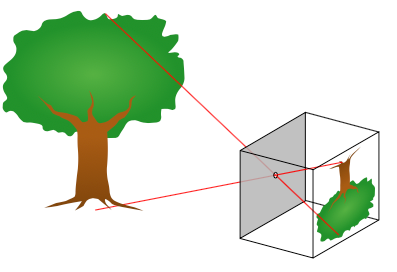###### Projection through a pinhole. (c) Wikimedia. User Pbroks13.

Mathematically and in the field of computer vision, points in three dimensional space are often denoted $$Q=[x,y,z] .$$ Their corresponding projection onto the camera image are $$q=[u,v,1] .$$ The '1' in $q$ is needed, as we are working with homogenous coordinates. For an explaination, click here. The two can be related by means of the pin-hole model:

$$q = \begin{bmatrix} f & 0 & c_x \\ 0 & f & c_y \\ 0 & 0 & 1 \end{bmatrix} \cdot Q$$###### The pinhole camera model. Modified from OpenCV online documentation. (c) Itseez.

This projection essentially does two things: it scales coordinates by $f$, and translates them such that coordinates are not relative to the camera center, but have a coordinate system that is located in the top left corner of the image. This is the natural way of indexing pixel positions in a digital image.

The parameters $c_x, c_y$ are called principle point coordinates as they can also be interpreted as the image-coordinates of the principle point, which is where the optical axis intersects the image plane. In m cameras, it is reasonable to assume that $c_x, c_y$ is at the exact image center. However, the lens may not be perfectly centered with the image sensor, in particular in low-cost cameras such as those in smartphones. And in specialized cameras and lenses such as Scheimpflug lenses or off-axis projection lenses, the optical axis does purposefully not intersect the image center. In these situations, $c_x, c_y$ need to be determined from calibration.

The parameter $f$ is the focal length, and it depends on the camera lens and sensor used. A large/long focal length scales by a large number and can be interpreted as a large "zoom", while a macro lens would have a small/short focal length. By units of $f$ necessarily have to be compatible with those of $q$ and $Q$. Hence, if 3d points $Q$ are given in meters and $q$ in pixels (or px), $f$ has units of $px/m$. Note that in practical applications, $Q$ is most often expressed in $m$ or $mm$, while $q$ would nearly always be in $px$. It is important, however, to keep in mind the position of the origin.

Extending the pinhole model

Through experimentation, and in order to accomodate cameras and lenses that are not well-described by means of the simple pinhole model, a common extension has the following parameters:

$$q = \begin{bmatrix} f_x & \alpha f_x & c_x \\ 0 & f_y & c_y \\ 0 & 0 & 1 \end{bmatrix} \cdot Q$$

The exact formulation differs a little from author to author. The important thing to note however, is that by introducing $f_x$ and $f_y$ instead of just one common $f$, we allow the camera to scale differently in $x$ and $y$ directions. The ratio $f_x/f_y$ is sometimes called the aspect ratio. This could be due to non-square pixels (seen in some digitization standards for analogue cameras, e.g. 480i or 576i), or some exotic anisotropic camera lenses. Depending on the quality of the camera that is being calibrated, including $f_x$ and $f_y$ may still be justified. Luckily, both parameters can be determined very robustly and repeatedly in camera calibration, and as such, there is usually not much harm done in calibrating for both.

$\alpha$ on the other hand models skew in the camera, which is rarely justified in practice in modern devices. It allows for the sensor's x- and y-axis to not be perfectly perpendicular. This parameter should be excluded from the camera model and calibration in almost all modern applications, unless good reason exist to include it, as otherwise, it makes calibration less robust.

Lens Distortion

The pin-hole model is only perfectly valid for a camera with an infinitely small entrace pupil (or aperture). In practice, such cameras do not exist of course, as they would not allow any light to enter. Hence, a system of lenses is usually required to focus the incoming rays onto the image sensor. In doing so, some degree of lens distortion is introduced. This is usually much more pronounced in wide angle macro lenses (sometimes called fish-eye lenses) than in long focal length lenses, because it is more difficult to construct wide-angle lenses with low distortion, even though manufacturers do their best to avoid it.

One group of lens distortion effects have radial symmetry, meaning that at a given distance from the principle point, the amount of distortion is constant. When the lens distorts inwards, this is termed barrel distortion. In contrast, when it disorts outwards, it is called pincushion distortion. A mixture of both is called handlebar distortion.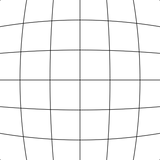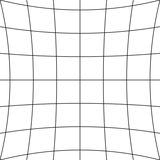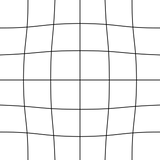###### Barrel distortion (left), pincushion distortion (middle) and mustache distortion (right). (c) Wikimedia. User WolfWings.

Beeing radially symmetric, a single function of variable $r$ is sufficient to describe the distortion effect. Using $u,v$ as image coordinates and $c_x, c_y$ beeing the principle point coordinates, we can define $r$:

$$r(u,v) = \sqrt{(u-c_x)^2 + (v-c_y)^2} ,$$

that is, $r(u,v)$ represents the distance from the image center.

Different suitable parametric function for radial lens distortion effects have been proposed in litterature. A widely accepted model is the even ordered radial model of Brown :

$$q_{\text{corrected}} = (1 + k_1 r^2 + k_2 r^4 + k_5 r^6) \cdot q .$$

In this model, three parameters $(k_1, k_2, k_5)$ are used. They are polynomial coefficient, allowing for a smootely varying distortion to be present, which can be strictly negative (barrel distortion), strictly positive (pincushion distortion) or both (mustache distortion). Note that only even polynomial orders need to be included as the function's domain is allways positive ($r \in [0; r_{\text{max}}]$).

Tangential Distortion Effects

An effect that is not readily explained with a radial distortion model is tangential or thin prism distortion, which can be due to decentering, ie. different lens elements not beeing perfectly aligned, or because the optical axis is not perfectly normal to the sensor plane. A useful model, which has been shown to fit the pixel distortion nicely was also proposed by Brown :

$$q_{\text{corrected}} = \begin{bmatrix} 2 k_3 x y + k_4 (r^2+2x^2) \\ k_3 (r^2 + 2*y^2) + 2 k_4 x y \end{bmatrix} \cdot q .$$

The combination of the radial model above and the tangential one is sometimes called the Plumb Bob model or Brown-Conrady model.

For good quality lenses and cameras, it is often not necessary to include tangential distortion parameters in the camera model. Just like central point coordinates and radial distortion parameters, a large amount of well distributed calibration images are usually needed to determine these parameters precisely and with good repeatiblity. As such, one is often better served by not including them, especially if the camera and lens are of sufficient quality.

The common way of representing points in 3d space or on a 2d plane is to use 3-vectors and 2-vectors respectively. However, for some purposes, it is very useful to use homogeneous coordinates instead, where an extra coordinate is introduced.

For instance, a 2-d image point may be denoted $q_{\text{inhom}}=[u,v]$. In homogeneous coordinates we write  $q_{\text{hom}}=s \cdot [u,v,1]$, and have $s$ as a free scale parameter. Any choice of $s$ represents the same 2-d point. However, we cannot allow $s$ to be 0 or infinity.

Now imagine a coordinate transformation consisting of scaling by a factor $\alpha$ and translating with a vector $[t_x, t_y]$. It could be applied to $q_{\text{inhom}}$ as follows:

$$\hat{q}_{\text{inhom}} = \begin{bmatrix} u \\ v \end{bmatrix} = \begin{bmatrix} \alpha & 0 \\ 0 & \alpha \end{bmatrix} q_{\text{inhom}} + \begin{bmatrix} t_x \\ t_y \end{bmatrix}$$

This does work well, however, the transformation is not linear (it cannot be expressed as a single matrix-vector product). For many derivations however, it would simplify the math greatly, if this transformation could indeed be expressed as a single matrix-vector operation. With the homogenous version of $q$, we can do the following:

$$\hat{q}_{\text{hom}} = s \begin{bmatrix} u \\ v \\ 1 \end{bmatrix} = \begin{bmatrix} \alpha & 0 & t_x \\ 0 & \alpha & t_y \\ 0 & 0 & 1 \end{bmatrix} q_{\text{hom}}$$

That is: now the transformation (a projection in this case) is expressed as a linear transformation. In order to convert $\hat{q}_{\text{hom}}$ to  $\hat{q}_{\text{inhom}}$, which we can readily interprete, we simply divide with the first two components of $\hat{q} _{\text{inhom}}$ with the third (which is the arbitrary scale factor $s$).

This trick allows many formulations for geometry to be expressed in a simpler way. In addition, there is a useful interpretation of homogenous 2d coordinates: We know that any point in a camera image corresponds to some point on a specific line in 3d space. The homogeneous coordinates for the 2d line are exactly the parameterization for this line in space. Varying $s$, we move along that line. Setting $s = 1$, we intersect the image plane and get the 2d point coordinates that we are usually interested in.

Homogenous coordinates have other nice properties. Wikipedia provides an excellent overview.

References

: Close-Range Camera Calibration, D.C. Brown, Photogrammetric Engineering, pages 855-866, Vol. 37, No. 8, 1971.]]>
https://calib.io/blogs/knowledge-base/calibration-patterns-explained 2018-11-15T16:35:00+01:00 2021-03-31T16:31:10+02:00 Calibration Patterns Explained Jakob W. Calibrating cameras accurately is important for the success of any machine/computer vision setup. However, there are different patterns to choose from. In order to make the selection easier for you, this article explains the main benefits of each.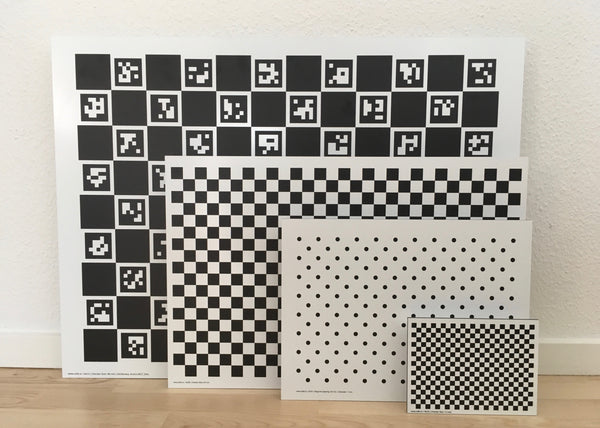###### A selection of the calibration patterns we offer at Calib.io. CharuCo, chessboard, asymmetric circles and chessboard.

Pattern size

In choosing a calibration plate, an important consideration is the physical size of it. This ultimately relates to the measurement field of view (FOV) of the final application. This is because cameras need to be focused on that specific distance and calibrated. Changing the focus distance slightly affects focal length, which would throw any previous calibration off. Even aperture changes usually have a negative effect on calibration validity, which is why they should be avoided.

For accurate calibration, the camera model is best constrained if the camera sees the calibration target filling most of the image. Popularity speaking, if a small calibration plate is used, many combinations of camera parameters could explain the observed images. As a rule of thumb, the calibration plate should have an area of at least half the available pixel area when observed frontally.

Pattern type

Different patterns have been introduced over the years, with each having unique properties and benefits.

Choosing the right type starts by considering which algorithm and algorithm implementation you will be using. In software such as Calib's Camera Calibrator and libraries such as libCalib, OpenCV or MVTec Halcon, there is some freedom regarding the pattern, and they have individual benefits and limitations.

Checkerboard targets

This is the most popular and common pattern design. Chessboard corners candidates are usually found by first binarizing the camera image and finding quadrilaterals (these are the black chessboard fields). A filtering step retains only those quads that meet certain size criteria and are organized in a regular grid structure whose dimensions match those specified by the user.

After an initial detection of the pattern, the corner locations can be determined with very high accuracy. This is because corners (mathematically: saddle points) are principally infinitely small and hence unbiased under perspective transformations or lens distortion.

In OpenCV, the entire chessboard must be visible in all images in order to be detected. This usually makes it difficult to obtain information from the very edges of images. These areas are usually good to have information from, as they constrain the lens distortion model properly.

Following the detection of a checkerboard, subpixel refinement can be performed to find the saddle points with subpixel accuracy. This makes use of the exact gray values of pixels around a given corner position, and the accuracy is much more accurate than what integer pixel positions would allow for.

An important detail regarding checkerboard targets is that in order to be rotation-invariant, the number of rows needs to be even and the number of columns odd, or the other way around. If for instance, both are even, there is a 180-degree rotation ambiguity. For single camera calibration, this is not an issue, but if the same points need to be identified by two or more cameras (for stereo calibration), this ambiguity must not exist. This is the reason why our standard checkerboard targets all have this property of even/odd rows/columns.

CharuCo targets

CharuCo patterns overcome some of the limitations of classical checkerboards. However, their detection algorithm is somewhat more complex. Luckily, CharuCo detection is part of OpenCVs contrib library (since OpenCV 3.0.0), making it very easy to integrate this advanced method.

The main advantage with CharuCo is that all light checker fields are uniquely coded and identifiable. This means that even partly occluded or non-ideal camera images can be used for calibration. For instance, strong ring lights may produce inhomogenous lighting on the calibration target (a region of semi-specular reflection), which would cause ordinary checkerboard detection to fail. With CharuCo, the remaining (good) saddle point detections can still be used. Saddle point localizations can be refined using subpixel detection just like checkerboards.

With observations close to image corners, this is an extremely useful property. As the target can be positioned such that the camera only sees it partly, we can gather information from the very edges and corners of the camera image. This usually leads to very good and robust determination of lens distortion parameters. For this reason, we highly recommend the use of CharuCo targets, when OpenCV 3.x is available.

Naturally, CharuCo targets can be used for stereo calibration. In this case, some code needs to be implemented to find those points that were detected in each camera individually, and those that were found in both (the intersection of points).

Checkerboard marker targets

These are based on traditional checkerboards, and can use the same detection algorithms. In addition, they contain three circles at their center, which allows for absolute referencing even with partial views of the checkerboard (as long as the circles are seen in all images). Hence, data from the image periphery can be included, which ensures that the fitted lens model is also valid in those parts of the image.

For many stereo calibration tasks, the checkerboard marker target brings all the benefits of a coded target such as ChArUco. It is supported in e.g. OpenCV 4.5+.

Circle grids

Circle girds are also a popular and very common calibration target design, based on circles, either with white circles on dark background or dark (black) circles on white background. In image processing terms, circles can be detected as "blobs" in the image. Some simple conditions on these binary blob regions, such as area, circularity, convexity, etc. can be applied to remove bad feature candidates. The

After finding suitable candidates, the regular structure of features is again used to identify and filter the pattern. The determination of circles can be done very accurately since all pixels on the periphery of circles can be used, decreasing the influence of image noise. However, in contrast to the saddle points in checkerboards, circles are imaged as ellipses under camera perspective. This perspective can be accounted for by means of image rectification. However, in addition, the unknown lens distortion means that the circles are not imaged as perfect ellipses, which adds a small bias. However, we can consider the distortion model as locally linear (obeying a perspective transformation / homography), so this error is very small in most lenses.

For high-accuracy calibration both the elliptical shape and the projected circle center need to be accounted for, especially with short focal length lenses and large circles. OpenCV does neither, and by default uses a simple blob detector to find the centroids of elliptical blobs. Calib Camera Calibrator does account for these effects and is able to produce more accurate results for circular targets.

An important difference between symmetric and asymmetric circle grids is that the former have a 180-degree ambiguity as explained in the "Checkerboard" section. Hence, for stereo calibration, asymmetric grids are necessary. Otherwise, there is not a big difference in the performance one should expect for either type.

]]>